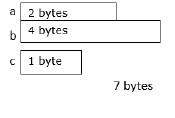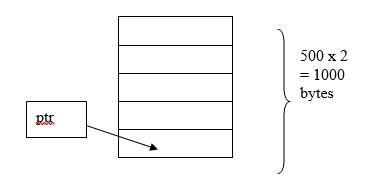# What is Calloc in C language?

The C library memory allocation function void *calloc(size_t nitems, size_t size) allocates the requested memory and returns a pointer to it.

The difference in malloc and calloc is that malloc does not set the memory to zero, whereas, calloc sets the allocated memory to zero.

## Memory allocation Functions

Memory can be allocated in two ways as explained below −Once memory is allocated at compile time, it cannot be changed during execution. There will be a problem of either insufficiency or else wastage of memory.

The solution is to create memory dynamically i.e. as per the requirement of the user during execution of program.

The standard library functions which are used for dynamic memory management are as follows −

• malloc ( )
• calloc ( )
• realloc ( )
• free ( )

## The Calloc ( ) function

• This function is used for allocating continuous blocks of memory at run time.

• This is specially designed for arrays.

• It returns a void pointer, which points to the base address of the allocated memory.

The syntax for calloc() function is given below −

void *calloc ( numbers of elements, size in bytes)

## Example

The following example shows the usage of calloc() function.

int *ptr;
ptr = (int * ) calloc (500,2);

Here, 500 blocks of memory each of size 2 bytes will be allocated continuously. Total memory allocated = 1000 bytes.int *ptr;
ptr = (int * ) calloc (n, sizeof (int));

## Example program

Given below is a C Program to compute sum of even numbers and odd numbers in a set of elements using dynamic memory allocation functions Calloc.

Live Demo

#include<stdio.h>
#include<stdlib.h>
void main(){
//Declaring variables, pointers//
int i,n;
int *p;
int even=0,odd=0;
//Declaring base address p using Calloc//
p = (int * ) calloc (n, sizeof (int));
printf("Enter the number of elements : ");
scanf("%d",&n);
/*Printing O/p -
We have to use if statement because we have to check if memory
has been successfully allocated/reserved or not*/
if (p==NULL){
printf("Memory not available");
exit(0);
}
//Storing elements into location using for loop//
printf("The elements are : ");
for(i=0;i<n;i++){
scanf("%d",p+i);
}
for(i=0;i<n;i++){
if(*(p+i)%2==0){
even=even+*(p+i);
} else {
odd=odd+*(p+i);
}
}
printf("The sum of even numbers is : %d",even);
printf("The sum of odd numbers is : %d",odd);
}

## Output

When the above program is executed, it produces the following result −

Enter the number of elements : 4
The elements are :
12
56
23
10
The sum of even numbers is : 78
The sum of odd numbers is : 23